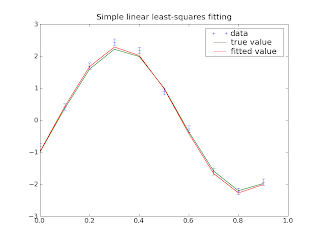### Curve fitting, part 1: linear least-squaresFitting a curve to data is a basic operation for many scientists. Usually it's a simple process, just use your favorite software to draw the best-fit line and forget it. But when you start having many points, large uncertainties, complicated curves, or (often) all three, it becomes more difficult. If you want to be able to say something statistical about the results, then you have to think carefully about the techniques you use. This post will just set up a simple curve fitting scenario and solve it using simple techniques. In future posts in this series I plan to add difficulties to the scenario and elaborations to the techniques, ultimately trying out some tools I've only read about.

I will set up and solve the problem in a literate programming style. The tools I use will be python, numpy, scipy, and matplotlib, but I hope that the code will be reasonably intelligible even if you're not familiar with the tools I'm using.
import numpy as npimport pylab as plt

First I will set up an object to represent the model. That is, it should be able to take a set of model parameters, and generate predicted average values for each data bin. It should also be able to generate "fake" data sets, which we will use to demonstrate our fitting (and, later, to analyze the quality).

The model I am using will be a linear combination of sine and cosine terms, and the measurements will be n equally-spaced measurements with equal uncertainty. (This particular model could be fit much more efficiently using a Fast Fourier Transform.)
class SinCosModel:  def __init__(self, n, uncertainty=1.):      self.initial_guess = np.array([1.,0.])      self.n = n      self.uncertainty = uncertainty  def predicted_values(self, parameters):      a, b = parameters      xs = np.arange(self.n)/float(self.n)      return a*np.sin(2*np.pi*xs) + b*np.cos(2*np.pi*xs)  def uncertainties(self, parameters):      return self.uncertainty*np.ones(self.n)  def generate(self, parameters):      return (self.predicted_values(parameters) +          np.random.normal(scale=self.uncertainty,size=self.n))

Now the fitting. Here I cheat: numpy provides a linear least-squares fitting procedure. It takes a linear system of the form Ax, b and adjusts the vector x to minimize the sum of squares of the values (Ax-b).
def fit_linear_least_squares(model, data):  n_params = len(model.initial_guess)  n_data = len(data)  assert len(model.predicted_values(model.initial_guess))==n_data

This is where the assumption of linearity is built in: I build the matrix A by evaluating the model at vectors like [1,0] and [0,1].
    coefficient_matrix = np.zeros((n_data,n_params))  for i in range(n_params):      params = np.zeros(n_params)      params[i] = 1      coefficient_matrix[:,i] = model.predicted_values(params)

Given that matrix, I use numpy to fit for the parameters. Numpy computes the least-squares fit using the singular value decomposition, a powerful technique in numerical linear algebra. This routine provides some control over the quality of the fit; in particular, the vector 's' contains information about the "singular values" of the linear fit. These will allow one to notice if roundoff error becomes a problem, which should only be the case if we have chosen parameters that have nearly the same effect ("degenerate"). In any case this does not tell us about the statistics of our fit, which is generally more of a concern (but which I will defer until the next article). So for our purposes the only value that's important is 'x', the vector of fitted parameters.
    x, residues, rank, s = np.linalg.lstsq(coefficient_matrix, data)  return x

Now a quick function to demo the curve fitting: it uses the model to generate a 'random' fake data set, fits a curve to it, and plots the true curve, the data, and the fitted curve.
def demo_linear_least_squares():  true_parameters = np.array([2.,-1.])  n = 10  uncertainty = 0.1  model = SinCosModel(n,uncertainty)  xs = np.arange(n)/float(n)  data = model.generate(true_parameters)  fit_parameters = fit_linear_least_squares(model, data)  plt.figure()  plt.errorbar(xs, data, uncertainty, label="data", fmt="+")  plt.plot(xs,model.predicted_values(true_parameters), label="true value")  plt.plot(xs,model.predicted_values(fit_parameters), label="fitted value")  plt.xlim(0,1)  plt.title("Simple linear least-squares fitting")  plt.legend(loc="best")  plt.savefig("linear-least-squares-1.png")

Finally, some code so that if you put all this in a file and run the file (e.g. as 'python curvefit.py') it will run the demo function and plot it to screen. The 'np.random.seed(0)' seeds the pseudorandom number generator so that the program generates the same output every time.
if __name__=='__main__':  np.random.seed(0)  demo_linear_least_squares()  plt.show()

This code produces the image seen at the top of this post: the fit is pretty good; any misfit is really forced by the data. But for real scientific data, we would want some quality checks: we would want uncertainties on the fitted values, and we would want some sort of measure of how well the curve fit the data. I'll talk about how to get these next time.